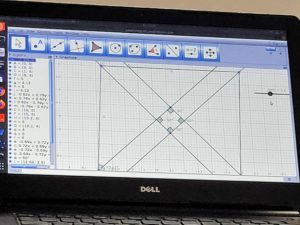## Dienes Block or Base 10 block or multi-base arithmetic blocks.

Zoltan Pal Dienes is the guy who invented these blocks. He was a Hungarian mathematician who believed that if we teach a subject like maths through games, play, and dance; chances that children will retain the information are greater than that of learning by traditional way using textbooks and lectures. He also created a six-stage theory to learn mathematics.

He was a tireless practitioner of ‘new mathematics’ and psycho mathematics (psychology of mathematics learning)

That’s why he came up with the idea of base 10 blocks, also known as Dienes blocks which help children understand numerical concepts like place value, number operations like addition, subtraction, multiplication, decimals, the square of a number. Or algebraic concepts like how to multiply two linear terms and how to find factors for algebraic expressions.

He also invented logic blocks which help children in understanding sets, differences, logical sequence, and classification.

Dienes block are an incredibly powerful manipulative. Can be used to enhance the understanding of fundamental maths topics such as place value and the four operations.

Base ten blocks are popular in elementary school mathematics instruction, especially with topics that students struggle with such as multiplication. They are frequently used in the classroom by teachers to model concepts, as well as by students to reinforce their own understanding of said concepts. Physically manipulating objects is an important technique used in learning basic mathematic principles, particularly at the early stages of cognitive development. Studies have shown that their use, like that of most mathematical manipulatives, decreases as students move into higher grades.

Students can use Dienes by manipulating them in several ways to show number and patterns. They are usually made of plastic or wood and come in four sizes which will indicate their place value.

• Units – one’s place
• Longs – ten’s place
• Flats – hundred’s place
• Blocks – thousand’s place

Multiplication of Dienes block

Dienes are important in school as they help students physically manipulate objects to learn basic principles. Being able to physically manipulate objects aids students’ early cognitive development.

## Li-Fi

LiFi, also known as “Light Fidelity” is a wireless optical networking technology, which uses light-emitting diodes (LEDs) to transmit data. In 2011, professor Harald Haas made a LiFi demonstration at the TED (Technology, Entertainment, Design) Global Talk on Visible Light Communication (VLC).

When an electrical current goes through to a LED light bulb, a stream of light (photons) emits from the lamp. LED bulbs are semiconductor devices, which means that the brightness of the light flowing through them can change at extremely high speeds. The signal is sent by modulating the light at different rates. The signal can then be received by a detector that interprets the changes in light intensity (the signal) as data. Also when the LED is ON, you transmit a digital 1, and when it is OFF, you transmit a 0.

From a laptop, the audio signal transmits through the light-emitting diode (LED) where the brightness of the light flowing through them can change at extremely high speeds. We used a solar panel as a receiver, the signal is amplified and sent as audio output in the speaker.

Video demonstrates how simple LiFi work’s.

## Fraction multiplication V2

From the STEMland scaling session with Swathi on Friday, the input received was to make the multiplication to be a perfect square to show that 1/4 is smaller than 1/3.

Murali from 9th grade implemented the change:

## Scratch Madala at Aikiyam school

Pratap

This week me and Murali went to Aikiyam to teach shapes using scratch. We started with the drawing a straight line. Then asked children to draw a triangle . Children took some time and then figured out the angle to draw a triangle. Then we asked them to draw the following shapes like square, pentagon, hexagon, heptagon , octagon. Then using this shapes we asked then to draw mandala. Children were interested to  draw their own mandalas . Children understood the concept of repeat loop. They were familiar with the  pen function in the scratch. Children were able to connect the angles for different shapes.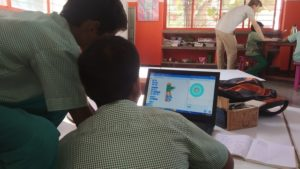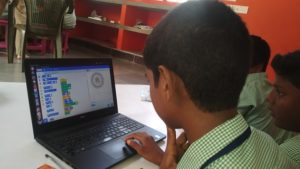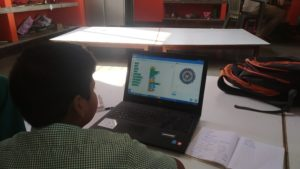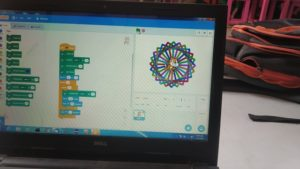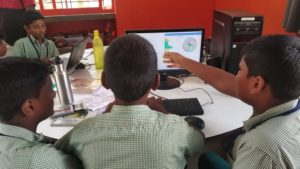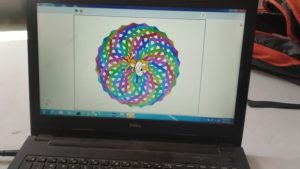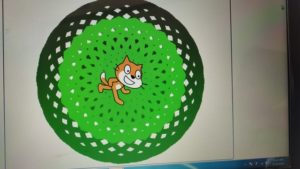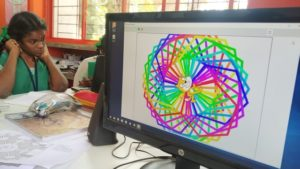## STEM land meeting with Swati Sircar

~Poovizhi, Murali and Arun

This week it was a large group of people in the meeting. Coordinators from Aikiyam, Udavi, Isai ambalam and AITI joined the meeting. Swati Sircar joined the meeting online. At first Sundar demonstrated Scratch projects on finding LCM created by 8th grade and program on Graph created by 9th grade children which asks the user to find the point and completes by drawing a bear. Then we listed few topics to discuss with Swati. Swati explained about Trignometry. She said we can ask children to come up with 6 possible ratios from a right angle triangle and map the names.

Then different methods of teaching multiplications

7×9 = 7×10-7

To explain and use multiplication in

1. Scaling
3. Cartesian product (Choices of combinations)
4. Area (rows and column)
5. Rate (price and qty)

Then we moved to Division and she explained how to spit 11/3. For example to draw three circles and draw apples in each circle equally and the remaining is the reminder.

Then we discussed about simplification. For simplification children need to learn divisibility rules for 2,3,4,5,9,10 and 11

Followed with we had a conversation on negative exponential

2^-x = 1/2^x

(1/2)^-5 = (2)^5

2^3 = 8

2^2 = 4

2^1 = 2

2^0 = 1

2^-1 = 1/2

2^-2 = 1/4

2^-3 = 1/8

## STEM land meeting with all STEM coordinators

~Murali and Poovizhi

STEM land meeting with all STEM coordinators

This  Friday (29/11/2019) we had an interesting sharing session. Abilash, Ranjith and Sivaraman presented an experiment on Electrolysis through which they generated hydrogen from water using salt and electricity. It was new for us and we learnt how oxygen and hydrogen splits from water.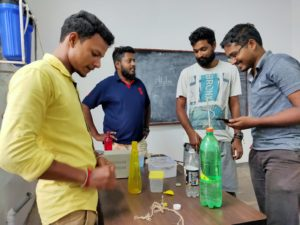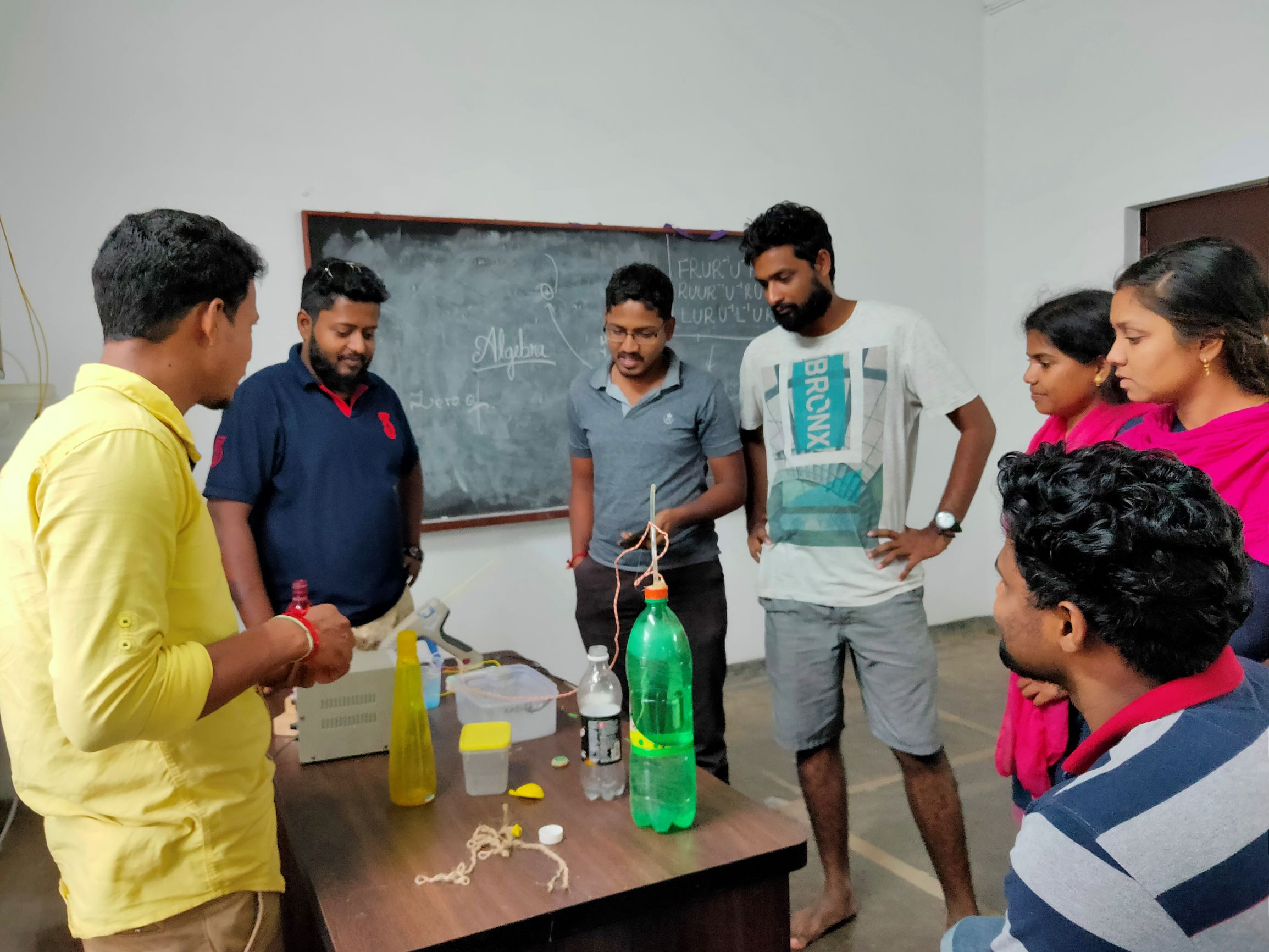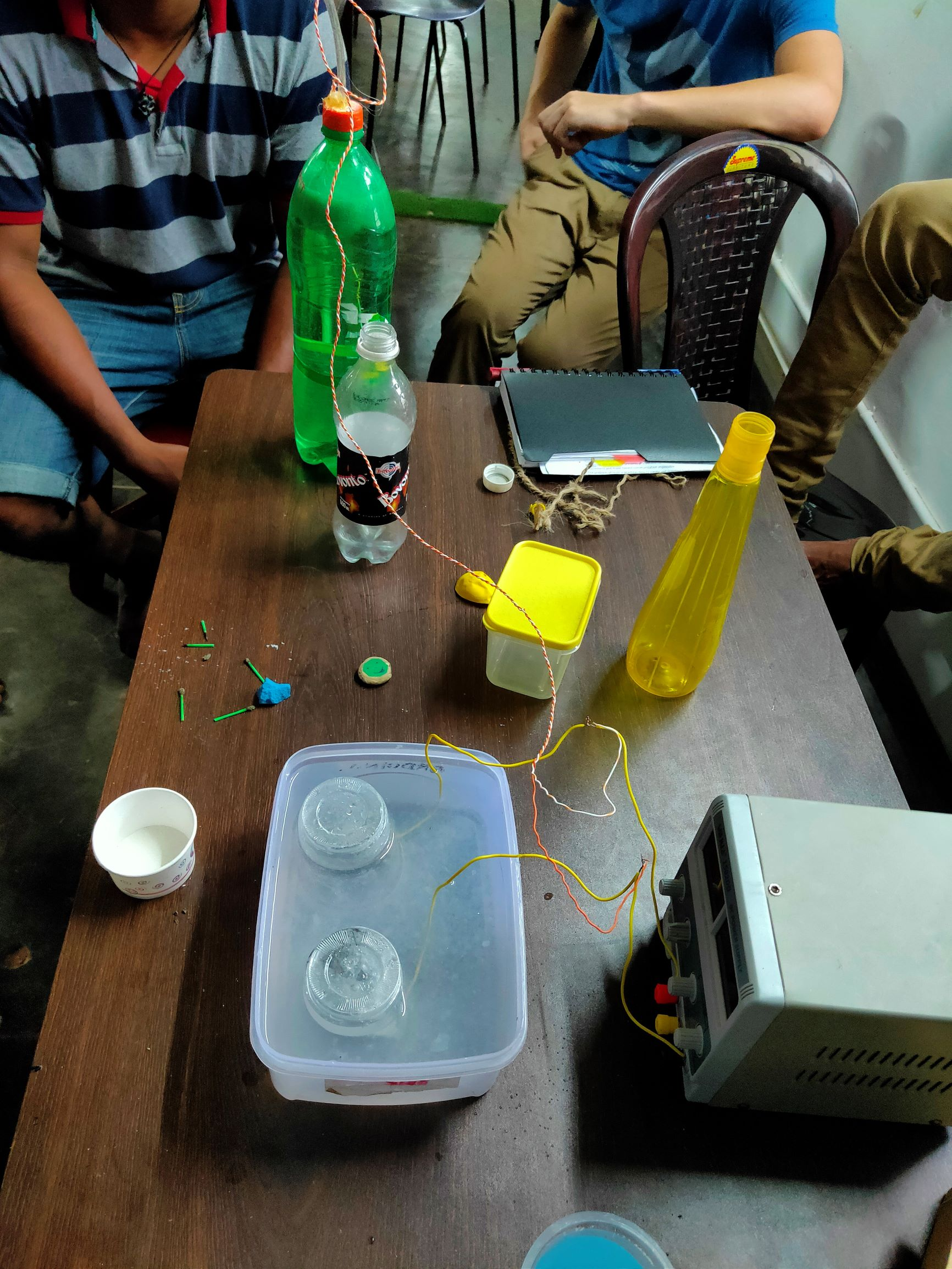After this practical experiment, Sundar from Udavi Stemland presented a scratch project on a graph in which the sprite moves to a particular point and asks the user to enter the (x,y) coordinates. Once the user enters the correct coordinates, it moves to the next point and so on. If the user completes until the end it draws an image.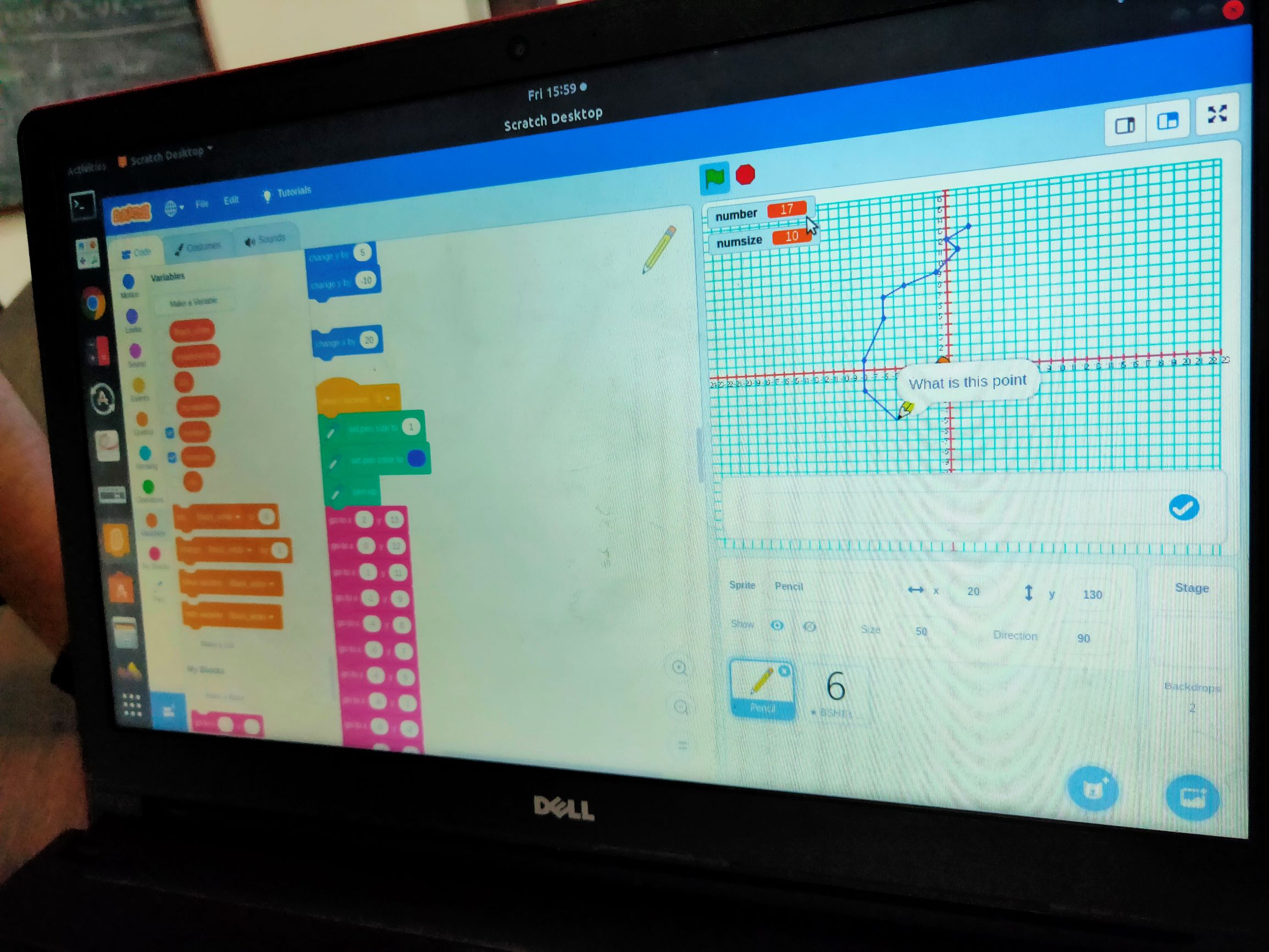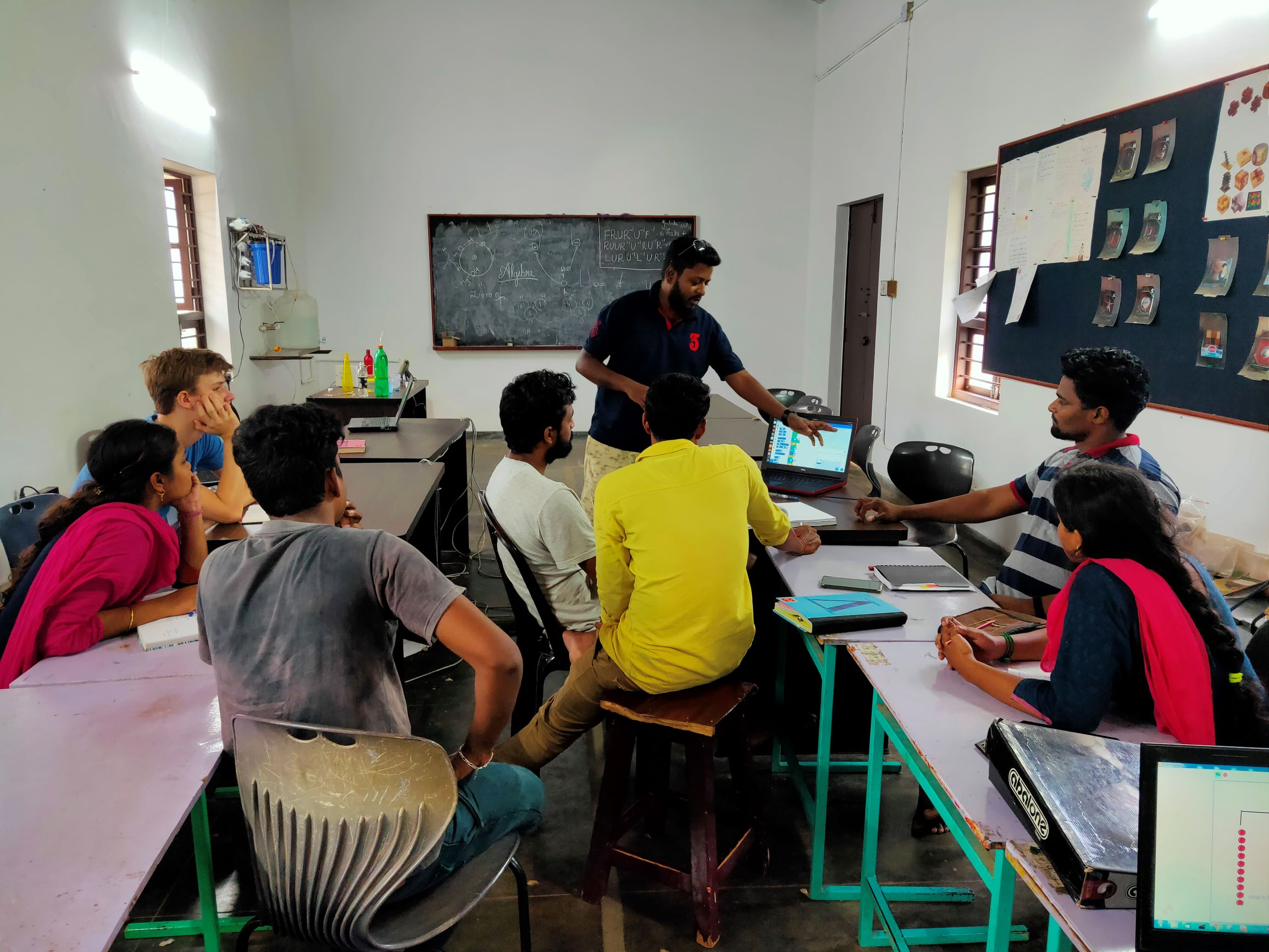Following this, we had another scratch project on algebraic expression. It is a game where the user is supposed to find the value of the given objects on both sides of a weighing scale.

## STEM land meeting with all STEM coordinators

~ Murali and Poovizhi

Sundar (Udavi school STEM land coordinator) gave a demonstration on learning Algebra using weighing scale. He used few sketch pens and weighing cubes (1gm) . For example one side had 10 grams and the other side had 5 sketch pens when both were weighing equal. He asked us to find the weight of each sketch pen. Then we discussed and solved few such examples in solveme and came up with all possible algebraic equations.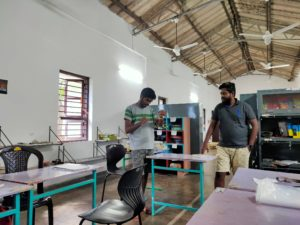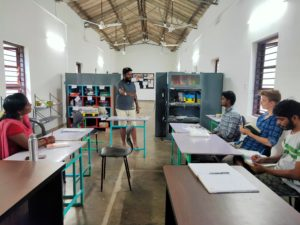Saravanan from Aikiyam school had brought a star tetrahedron in which children had learnt about pyramids and triangles.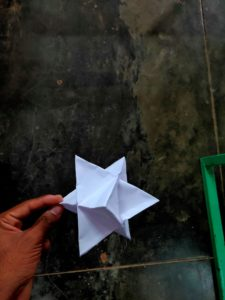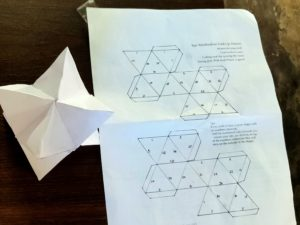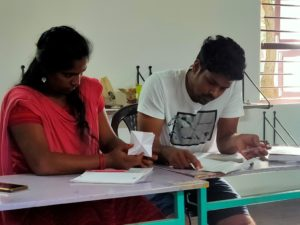Arun who is coordinating with AIIT explained about parallelogram and showed how a rectangle can be formed by bisecting the angles of the parallelogram.